Author: Oscar Cronquist Article last updated on January 17, 2020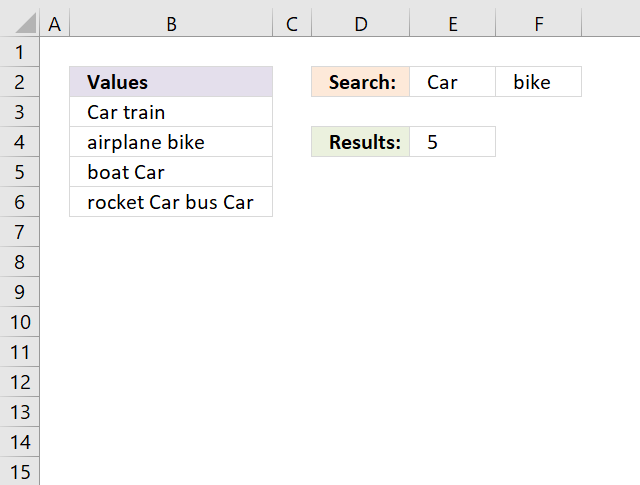This article demonstrates an array formula that counts how many times multiple text strings exist in a cell range.  The formula takes into account if a text string exists multiple times in a single cell, see picture above. Text strings "Car" and "bike" exist 5 times in cell range B3:B6.

It is easy to add more text strings, adjust cell range E2:F2 in the formula. The following formulas are case sensitive, however I will be demonstrating case insensitive formulas later in this article.

Array formula in cell E4:

=SUM((LEN(B3:B6)-LEN(SUBSTITUTE(B3:B6,E2:F2,"")))/LEN(E2:F2))

or use this slightly larger regular formula:

=SUM(INDEX((LEN(B3:B6)-LEN(SUBSTITUTE(B3:B6,E2:F2,"")))/LEN(E2:F2),0,0))

### How to enter an array formula

1.  Select cell E4.
2. Press with left mouse button on in the formula bar.3. Copy above array formula and paste to the formula bar.
4. Press and hold CTRL + SHIFT simultaneously.
5. Press Enter once.
6. Release all keys.

The formula bar displays curly brackets:

{=SUM((LEN(A2:A5)-LEN(SUBSTITUTE(A2:A5,D1:E1,"")))/LEN(D1:E1))}

Don't enter the curly brackets yourself, they appear automatically.

### Explaining array formula in cell D2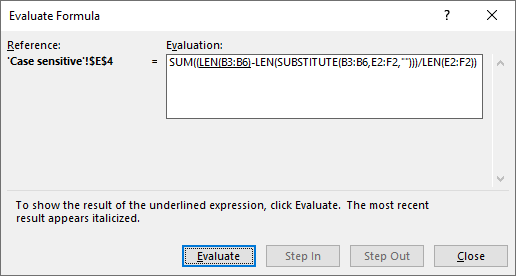Excel has a built-in tool named "Evaluate Formula" tool, it allows you to examine formulas and the calculations they make step by step.

Go to tab "Formulas" on the ribbon, press with left mouse button on "Evaluate Formula" button and a dialog box appears.

Press with left mouse button on the "Evaluate" button on the dialog box to move to the next calculation step, this way you can see all calculation steps a formula does making it easier for you to understand and troubleshoot a formula.

The most important thing to understand in this formula is how arrays can be used.

#### Step 1 - Count characters in each cell

The LEN function returns a number representing the number of characters in a cell. In this case, the LEN function works with a cell range returning an array of numbers.

Len(B3:B6)

becomes

Len({"Car train"; "airplane bike"; "boat Car"; "rocket Car bus Car"})

and returns {9; 13; 8; 18}.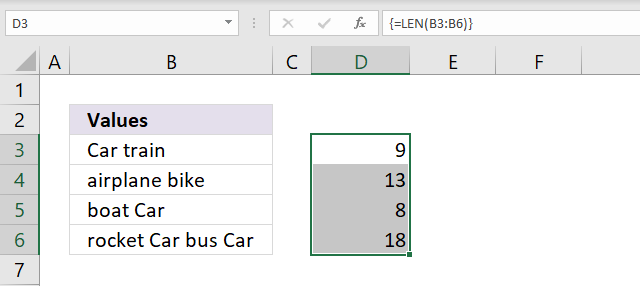#### Step 2 - Replace existing text with the new text string

The SUBSTITUTE function replaces a specific text string in a value, it is case sensitive. The SUBSTITUTE function replaces multiple strings with nothing in this formula.

SUBSTITUTE(text, old_text, new_text, [instance_num])

The old_text argument allows you to use a cell range D1:E1 which is handy in this scenario, note that it returns an array containing eight values.

The first row in the array contains values without the first text string (D1) and the second row contains values without the second text string (E1). This is not a problem as you will see when we go through the remaining steps.

SUBSTITUTE(B3:B6,D1:E1,"")

becomes

SUBSTITUTE({"Car train"; "airplane bike"; "boat Car"; "rocket Car bus Car"},{"Car", "bike"},"")

and returns {" train", "Car train"; "airplane bike", "airplane "; "boat ", "boat Car"; "rocket  bus ", "rocket Car bus Car"}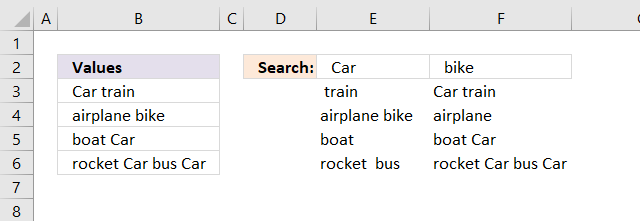Values in an array are separated by a comma or a semicolon, commas are used between columns and semicolons between rows.

Note, your Excel version may use other characters than commas and semicolons based on the regional settings on your computer.

#### Step 3 - Count characters in each cell

In this step the LEN function calculates the length of each value in the array after the SUBSTITUTE function has replaced the given text strings.

LEN(SUBSTITUTE(B3:B6, E2:F2, ""))

becomes

LEN({" train", "Car train"; "airplane bike", "airplane "; "boat ", "boat Car"; "rocket  bus ", "rocket Car bus Car"})

and returns {6, 9; 13, 9; 5, 8; 12, 18}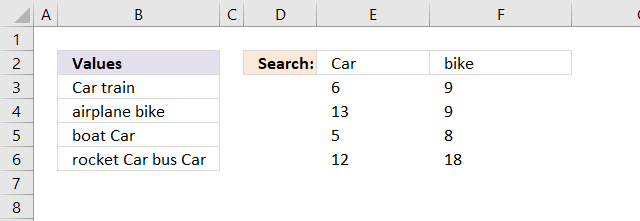#### Step 4 - Subtract original character length with substituted values

This step calculates the difference between the number of characters of each value in the array. But the array sizes do not match? The number of horizontal values matches which makes it possible to calculate this arithmetic operation.

LEN(B3:B6)-LEN(SUBSTITUTE(B3:B6,E2:F2,""))

becomes

{9; 13; 8; 18} - {6, 9; 13, 9; 5, 8; 12, 18}

an returns

{3, 0; 0, 4; 3, 0; 6, 0}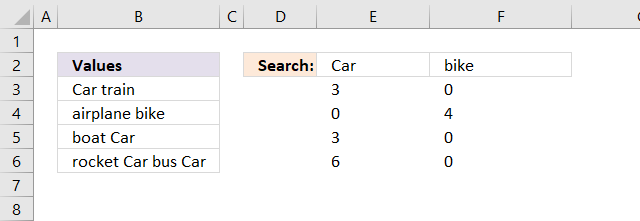#### Step 5 - Divide with length of each search string

We now know where the given text strings are in the array and also how many they are in each value based on character length. If we divide the length of each value in the array with the numbers of characters in each given text string we can calculate how many times they exist.

(LEN(B3:B6)-LEN(SUBSTITUTE(B3:B6,E2:F2,"")))/LEN(E2:F2)

becomes

{3,0;0,4;3,0;6,0}/LEN(D1:E1)

becomes

{3,0;0,4;3,0;6,0}/{3,4}

and returns {1, 0; 0, 1; 1, 0; 2, 0}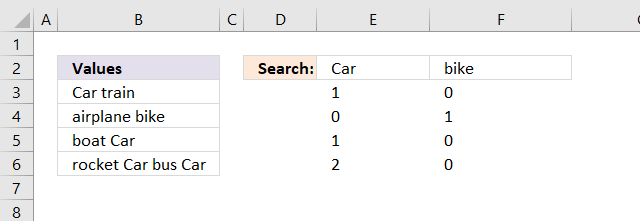#### Step 6 - Sum all values

The SUM function adds all numbers in the array.

SUM((LEN(B3:B6)-LEN(SUBSTITUTE(B3:B6,E2:F2,"")))/LEN(E2:F2)

becomes

SUM({1,0;0,1;1,0;2,0})

and returns 5 in cell E4.### Case insensitive counting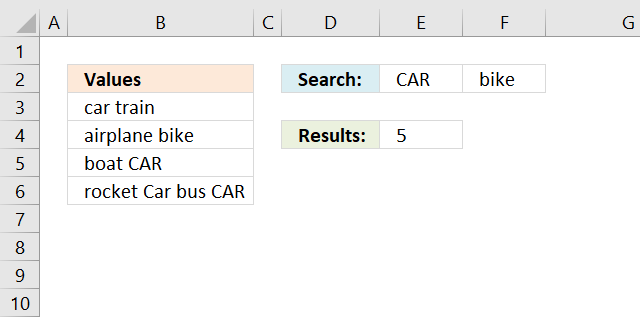The following formulas are not case sensitive in terms of search values, see image above.

Array formula in cell E4:

=SUM((LEN(B3:B6)-LEN(SUBSTITUTE(UPPER(B3:B6), UPPER(E2:F2), "")))/LEN(E2:F2))

or use this slightly larger regular formula:

=SUM(INDEX((LEN(B3:B6)-LEN(SUBSTITUTE(UPPER(B3:B6), UPPER(E2:F2),"")))/LEN(E2:F2), 0, 0))

The difference between these formulas and the case sensitive formulas is the UPPER function. It converts letters to upper case letters.

### Get the Excel fileCount-multiple-text-strings-in-a-cell-rangev2.xlsx